Updating search results...

# 235 Results

View
Selected filters:
• CalculusConditional Remix & Share Permitted
CC BY-NC-SA
Rating
0.0 stars

Using Green's Theorem to establish a two dimensional version of the Divergence Theorem

Subject:
Calculus
Mathematics
Material Type:
Lesson
Provider:
Author:
Sal Khan
02/14/2017Conditional Remix & Share Permitted
CC BY-NC-SA
Rating
0.0 stars

Intuition behind the Divergence Theorem in three dimensions

Subject:
Calculus
Mathematics
Material Type:
Lesson
Provider:
Author:
09/22/2013Conditional Remix & Share Permitted
CC BY-NC-SA
Rating
0.0 stars

Using the first and second derivatives to identify critical points and inflection points and to graph the function.

Subject:
Calculus
Mathematics
Material Type:
Lesson
Provider:
Author:
Sal Khan
09/23/2013Conditional Remix & Share Permitted
CC BY-NC-SA
Rating
0.0 stars

This video is from the Khan Academy subject of Math on the topic of Calculus and it covers Antiderivative of hairier expression.

Subject:
Calculus
Mathematics
Material Type:
Lesson
Provider:
Author:
04/14/2016Conditional Remix & Share Permitted
CC BY-NC-SA
Rating
0.0 stars

This video is from the Khan Academy subject of Math on the topic of Calculus and it covers Antiderivative of xcosx using integration by parts.

Subject:
Calculus
Mathematics
Material Type:
Lesson
Provider:
Author:
Rishi Desai
09/22/2013Conditional Remix & Share Permitted
CC BY-NC-SA
Rating
0.0 stars

This video is from the Khan Academy subject of Math on the topic of Calculus and it covers Antiderivatives and indefinite integrals.

Subject:
Calculus
Mathematics
Material Type:
Lesson
Provider:
Author:
Monterey Institute for Technology and Education
Sal Khan
09/22/2013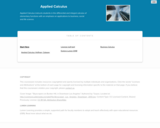Some Rights Reserved
Rating
0.0 stars

Applied Calculus instructs students in the differential and integral calculus of elementary functions with an emphasis on applications to business, social and life science. Different from a traditional calculus course for engineering, science and math majors, this course does not use trigonometry, nor does it focus on mathematical proofs as an instructional method.

Subject:
Calculus
Mathematics
Material Type:
Full Course
Textbook
Provider:
Lumen Learning
Provider Set:
Candela Courseware
Author:
Dale Hoffman
David Lippman
Shana Calaway
01/12/2021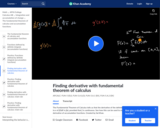Conditional Remix & Share Permitted
CC BY-NC-SA
Rating
0.0 stars

This video is from the Khan Academy subject of Math on the topic of Calculus and it covers Applying the fundamental theorem of calculus.

Subject:
Calculus
Mathematics
Material Type:
Lesson
Provider:
Author:
Sal Khan
11/16/2016Conditional Remix & Share Permitted
CC BY-NC-SA
Rating
0.0 stars

This video is from the Khan Academy subject of Math on the topic of Calculus and it covers Applying the product rule for derivatives.

Subject:
Calculus
Mathematics
Material Type:
Lesson
Provider:
Author:
Sal Khan
09/22/2013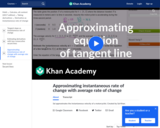Conditional Remix & Share Permitted
CC BY-NC-SA
Rating
0.0 stars

This video is from the Khan Academy subject of Math on the topic of Calculus and it covers Approximating equation of tangent line word problem.

Subject:
Calculus
Mathematics
Material Type:
Lesson
Provider:
Author:
04/14/2016Conditional Remix & Share Permitted
CC BY-NC-SA
Rating
0.0 stars

This video is from the Khan Academy subject of Math on the topic of Calculus and it covers Approximating instantaneous rate of change word problem.

Subject:
Calculus
Mathematics
Material Type:
Lesson
Provider:
Author:
Sal Khan
09/22/2013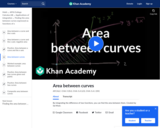Conditional Remix & Share Permitted
CC BY-NC-SA
Rating
0.0 stars

This video is from the Khan Academy subject of Math on the topic of Calculus and it covers Area between curves.

Subject:
Calculus
Mathematics
Material Type:
Lesson
Provider:
Author:
09/22/2013Conditional Remix & Share Permitted
CC BY-NC-SA
Rating
0.0 stars

This video is from the Khan Academy subject of Math on the topic of Calculus and it covers Area between curves with multiple boundaries.

Subject:
Calculus
Mathematics
Material Type:
Lesson
Provider:
Author:
09/22/2013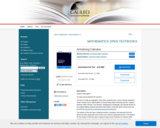Conditional Remix & Share Permitted
CC BY-NC-SA
Rating
0.0 stars

The text is mostly an adaptation of two other excellent open- source calculus textbooks: Active Calculus by Dr. Matt Boelkins of Grand Valley State University and Drs. Gregory Hartman, Brian Heinold, Troy Siemers, Dimplekumar Chalishajar, and Jennifer Bowen of the Virginia Military Institute and Mount Saint Mary's University. Both of these texts can be found at http://aimath.org/textbooks/approved-textbooks/.
The authors of this text have combined sections, examples, and exercises from the above two texts along with some of their own content to generate this text. The impetus for the creation of this text was to adopt an open-source textbook for Calculus while maintaining the typical schedule and content of the calculus sequence at our home institution.

Subject:
Calculus
Mathematics
Material Type:
Textbook
Provider:
University System of Georgia
Provider Set:
Galileo Open Learning Materials
Author:
Jared Schlieper,
Michael Tiemeyer
03/20/2015Conditional Remix & Share Permitted
CC BY-NC-SA
Rating
0.0 stars

This video is from the Khan Academy subject of Math on the topic of Calculus and it covers Basic trig and exponential antiderivatives.

Subject:
Calculus
Mathematics
Material Type:
Lesson
Provider:
Author:
Rishi Desai
09/22/2013Conditional Remix & Share Permitted
CC BY-NC-SA
Rating
0.0 stars

This video is from the Khan Academy subject of Math on the topic of Calculus and it covers Both bounds being a function of x.

Subject:
Calculus
Mathematics
Material Type:
Lesson
Provider:
Author:
Sal Khan
09/22/2013Conditional Remix & Share Permitted
CC BY-NC-SA
Rating
0.0 stars

This video is from the Khan Academy subject of Math on the topic of Calculus and it covers Building the idea of epsilon-delta definition.

Subject:
Calculus
Mathematics
Material Type:
Lesson
Provider:
Author:
CK-12 Foundation
Sal Khan
11/16/2016Some Rights Reserved
Rating
0.0 stars

This course provides an introduction to applied concepts in Calculus that are relevant to the managerial, life, and social sciences. Students should have a firm grasp of the concept of functions to succeed in this course. Topics covered include derivatives of basic functions and how they can be used to optimize quantities such as profit and revenues, as well as integrals of basic functions and how they can be used to describe the total change in a quantity over time.

Subject:
Calculus
Mathematics
Material Type:
Full Course
Textbook
Provider:
Lumen Learning
Provider Set:
Candela Courseware
01/12/2021Unrestricted Use
CC BY
Rating
0.0 stars

MATH&148 is a calculus course for business students. It is designed for students who want a brief course in calculus. Topics include differential and integral calculus of elementary functions. Problems emphasize business and social science applications. Translating words into mathematics and solving word problems are emphasized over algebra. Applications are mainly business oriented (e.g. cost, revenue, and profit). Mathematical theory and complex algebraic manipulations are not mainstays of this course, which is designed to be less rigorous than the calculus sequence for scientists and engineers. Topics are presented according to the rule of four: geometrically, numerically, analytically, and verbally. That is, symbolic manipulation must be balanced with graphical interpretation, numerical examples, and writing. Trigonometry is not part of the course.

Subject:
Calculus
Mathematics
Material Type:
Assessment
Full Course
Syllabus
Textbook
Provider:
Washington State Board for Community & Technical Colleges
Provider Set:
Open Course Library
01/13/2021Conditional Remix & Share Permitted
CC BY-NC-SA
Rating
0.0 stars

Let's calculate the integral from the last video.

Subject:
Calculus
Mathematics
Material Type:
Lesson
Provider: